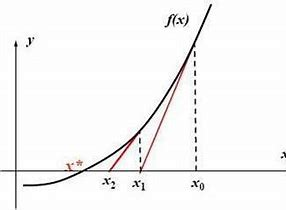导数的应用

相关变化率

1. 对涉及变化率的问题进行建模
2. 变量对$$t$$进行求导，$$\frac{\mathrm{d}Q}{\mathrm{d}t}$$使用已知条件v(变化率)进行替换
3. 对方程进行求解变量之间的相关变化率

中值定理

$f'(c)=0$

$f'(c)=\frac{f(b)-f(a)}{b-a}$

Proof:

let $$g(x)=f(x)-\frac{f(b)-f(a)}{b-a}(x-a)$$

that $$g(a)=g(b)=f(a)$$

According to Rolle Theorem

There is c let $$g'(x)=0$$

which is

$g'(x)=f'(x)-\frac{f(b)-f(a)}{b-a}\\ f'(c)=\frac{f(b)-f(a)}{b-a}$

最优化

1. 分析问题，数学建模
2. 求导使用函数极值解决最优化问题

线性化

$f(a+\Delta x) \approx f(a)+f'(a)\Delta x$Newton法

Newton法

$$a$$为$$f(x)=0$$的近似解

$$b=a-\frac{f(a)}{f'(a)}$$ $$b$$为更优解